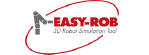EASY-ROB™ Kernel  v8.603
DFRAME Struct Reference

Homogeneous 4x4 transformation matrix, a Frame
with 3x3 orthogonal noa-matrix (n = o x a) and 3x1 position vector. The last row is per default [0 0 0 1]. More...

`#include <erk_capi_types.h>`

## Public Attributes

double n 
normal vector, n = o x a More...

double o 
orientation vector, o = a x n More...

double a 
approach vector, a = n x o More...

double p 
position vector More...

## Detailed Description

Homogeneous 4x4 transformation matrix, a Frame
with 3x3 orthogonal noa-matrix (n = o x a) and 3x1 position vector. The last row is per default [0 0 0 1].

## ◆ a

 double DFRAME::a

approach vector, a = n x o

## ◆ n

 double DFRAME::n

normal vector, n = o x a

## ◆ o

 double DFRAME::o

orientation vector, o = a x n

## ◆ p

 double DFRAME::p

position vector

The documentation for this struct was generated from the following file: